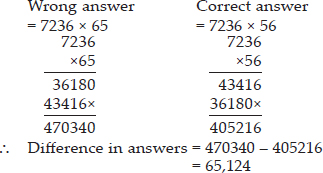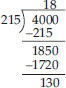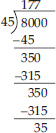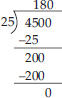Knowing Our Numbers (Mathematics) Class 6 - NCERT Questions
Q 1.

Fill in the blanks:
(A) 1 lakh = _______ ten thousand.
(B) 1 million = _______ hundred thousand.
(C) 1 crore = _______ ten lakh.
(D) 1 crore = _______ million.
(E) 1 million = _______ lakh.

SOLUTION:

(A) 10 : 1 lakh = 1,00,000
= 10 × 10,000 = 10 ten thousand
(B) 10 : 1 million = 1,000,000
= 10 × 100,000 = 10 hundred thousand
(C) 10 : 1 crore = 1,00,00,000
= 10 × 10,00,000 = 10 ten lakh
(D) 10 : 1 crore = 10 million
(E) 10 : 1 million = 10 lakh

Q 2.

Place commas correctly and write the numerals:
(A) Seventy three lakh seventy five thousand three hundred seven.
(B) Nine crore five lakh forty one.
(C) Seven crore fifty two lakh twenty one thousand three hundred two.
(D) Fifty eight million four hundred twenty three thousand two hundred two.
(E) Twenty three lakh thirty thousand ten.

SOLUTION:

(A) 73,75,307
(B) 9,05,00,041
(C) 7,52,21,302
(D) 58,423,202
(E) 23,30,010

Q 3.

Insert commas suitably and write the names according to Indian System of Numeration :
(A) 87595762
(B) 8546283
(C) 99900046
(D) 98432701

SOLUTION:

(A) 8,75,95,762 → Eight crore seventy-five lakh ninety-five thousand seven hundred sixty-two.
(B) 85,46,283 → Eighty-five lakh forty-six thousand two hundred eighty-three.
(C) 9,99,00,046 → Nine crore ninety-nine lakh forty-six.
(D) 9,84,32,701 → Nine crore eighty-four lakh thirty-two thousand seven hundred one.

Q 4.

Insert commas suitably and write the names according to International System of Numeration:
(A) 78921092
(B) 7452283
(C) 99985102
(D) 48049831

SOLUTION:

(A) 78,921,092 → Seventy-eight million nine hundred twenty-one thousand ninety-two
(B) 7,452,283 → Seven million four hundred fifty-two thousand two hundred eighty-three
(C) 99,985,102 → Ninety-nine million nine hundred eighty-five thousand one hundred two
(D) 48,049,831 → Forty-eight million forty-nine thousand eight hundred thirty-one

Q 5.

A book exhibition was held for four days in a school. The number of tickets sold at the counter on the first, second, third and final day was respectively 1094, 1812, 2050 and 2751. Find the total number of tickets sold on all the four days.

SOLUTION:

Number of tickets sold on first day = 1,094
Number of tickets sold on second day = 1,812
Number of tickets sold on third day = 2,050
Number of tickets sold on fourth day = 2,751
Total tickets sold = 1,094 + 1,812 + 2,050 + 2,751
= 7,707
Therefore, 7,707 tickets were sold on all the four days.

Q 6.

Shekhar is a famous cricket player. He has so far scored 6980 runs in test matches. He wishes to complete 10,000 runs. How many more runs does he need?

SOLUTION:

Number of runs to achieve = 10,000
Number of runs scored = 6,980
Number of runs required = 10,000 - 6,980 = 3,020
Therefore, Shekhar needs 3,020 more runs.

Q 7.

In an election, the successful candidate registered 5,77,500 votes and his nearest rival secured 3,48,700 votes. By what margin did the successful candidate win the election?

SOLUTION:

Number of votes secured by successful candidate = 5,77,500
Number of votes secured by his nearest rival = 3,48,700
Margin between them = 5,77,500 - 3,48,700
= 2,28,800
Therefore, the successful candidate won by a margin of 2,28,800 votes.

Q 8.

Kirti bookstore sold books worth Rs 2,85,891 in the first week of June and books worth
Rs 4,00,768 in the second week of the month. How much was the sale for the two weeks together? In which week was the sale greater and by how much?

SOLUTION:

Worth of books sold in first week = Rs 2,85,891
Worth of books sold in second week = Rs 4,00,768
Total worth of books sold = Rs (2,85,891 + 4,00,768)
= Rs 6,86,659
Since, 4,00,768 > 2,85,891
Therefore, sale of second week is greater than that of first week by Rs (4,00,768 - 2,85,891)
= Rs 1,14,877

Q 9.

Find the difference between the greatest and the least number that can be written using the digits 6, 2, 7, 4, 3 each only once.

SOLUTION:

Greatest five-digit number using digits
6,2,7,4,3 = 76432
Smallest five-digit number using digits
6,2,7,4,3 = 23467
Difference = 76432 - 23467 = 52965
Therefore, the difference is 52,965.

Q 10.

A machine, on an average, manufactures 2,825 screws a day. How many screws did it produce in the month of January 2006?

SOLUTION:

Number of screws manufactured in one day = 2,825
Number of screws manufactured in the month of January (31 days) = 2,825 × 31 = 87,575
Therefore, the machine produced 87,575 screws in the month of January.

Q 11.

A merchant had Rs 78,592 with her. She placed an order for purchasing 40 radio sets at Rs 1200 each. How much money will remain with her after the purchase?

SOLUTION:

Cost of one radio set = Rs 1200
Cost of 40 radio sets = Rs (1200 × 40) = Rs 48,000
Now, total money with merchant = Rs 78,592
Money left with her = Rs (78,592 - 48,000)
= Rs 30,592
Therefore, Rs 30,592 will remain with her after the purchase.

Q 12.

A student multiplied 7236 by 65 instead of multiplying by 56. By how much was his answer greater than the correct answer? (Hint: Do you need to do both the multiplications?)

SOLUTION:Q 13.

To stitch a shirt, 2 m 15 cm cloth is needed. Out of 40 m cloth, how many shirts can be stitched and how much cloth will remain?
(Hint: convert data in cm.)

SOLUTION:

Cloth required to stitch one shirt
= 2 m 15 cm = 2 × 100 cm + 15 cm = 215 cm
Length of cloth = 40 m = 40 × 100 cm = 4000 cm
Number of shirts can be stitched = 4000 ÷ 215Therefore, 18 shirts can be stitched and 130 cm (1 m 30 cm) cloth will remain.

Q 14.

Medicine is packed in boxes, each weighing 4 kg 500 g. How many such boxes can be loaded in a van which cannot carry beyond 800 kg?

SOLUTION:

The weight of one box = 4 kg 500 g
= 4 × 1000 g + 500 g = 4500 g
= 800 kg = 800 × 1000 g = 800000 g
Number of boxes = 800000 ÷ 4500Therefore, 177 boxes can be loaded in the van.

Q 15.

The distance between the school and the house of a student is 1 km 875 m. Everyday she walks both ways. Find the total distance covered by her in six days.

SOLUTION:

Distance between the school and house
= 1 km 875 m = 1 km + 875/1000 km = 1.875 km
Total distance covered in one day
= (1.875 × 2)km = 3.750 km
Distance covered in six days = (3.750 × 6) km
= 22.500 km
Therefore, a student covered 22 km 500 m distance in six days.

Q 16.

A vessel has 4 litres and 500 ml of curd. In how many glasses, each of 25 ml capacity, can it be filled?

SOLUTION:

Quantity of curd in the vessel
= 4 litres 500 ml = 4 × 1000 ml + 500 ml
= 4500 ml
Capacity of one glass = 25 ml
Number of glasses can be filled = 4500 ÷ 25Therefore, 180 glasses can be filled by curd.

Q 17.

Estimate each of the following using general rule:
(A) 730 + 998
(B) 796 - 314
(C) 12,904 + 2,888
(D) 28,292 - 21,496
Make ten more such examples of addition, subtraction and estimation of their outcome.

SOLUTION:

(A) 730 rounds off to 700
998 rounds off to 1,000
∴ Estimated sum = 700 + 1,000 = 1,700
(B) 796 rounds off to 800
314 rounds off to 300
∴ Estimated difference = 800 - 300 = 500
(C) 12,904 rounds off to 13,000
2,888 rounds off to 3,000
∴ Estimated sum = 13,000 + 3,000 = 16,000
(D) 28,292 rounds off to 28,000
21,496 rounds off to 21,000
∴ Estimated difference = 28,000 - 21,000
= 7,000
Ten more examples:
(I) 540 + 868
540 rounds off to 500
868 rounds off to 900
∴ Estimated sum = 500 + 900 = 1,400
(ii) 1,369 + 215
1,369 rounds off to 1,000
215 rounds off to 200
∴ Estimated sum = 1,000 + 200 = 1,200
(iii) 46,352 - 11,867
46,352 rounds off to 46,000
11,867 rounds off to 12,000
∴ Estimated difference = 46,000 - 12,000
= 34,000
(iv) 14,902 + 6,565
14,902 rounds off to 15,000
6,565 rounds off to 7,000
∴ Estimated sum = 15,000 + 7,000 = 22,000
(v) 514 - 386
514 rounds off to 500
386 rounds off to 400
∴ Estimated difference = 500 - 400 = 100
(vi) 27,904 + 69,592
27,904 rounds off to 28,000
69,592 rounds off to 70,000
∴ Estimated sum = 28,000 + 70,000 = 98,000
(vii) 530 - 98
530 rounds off to 500
98 rounds off to 100
∴ Estimated difference = 500 - 100 = 400
(viii) 18,230 - 3,666
18,230 rounds off to 18,000
3,666 rounds off to 4,000
∴ Estimated difference = 18,000 - 4,000
= 14,000
(ix) 56,306 + 17,693
56,306 rounds off to 56,000
17,693 rounds off to 18,000
∴ Estimated sum = 56,000 + 18,000 = 74,000
(x) 4,275 - 125
4,275 rounds off to 4,000
125 rounds off to 100
∴ Estimated difference = 4,000 - 100 = 3,900

Q 18.

Give a rough estimate (by rounding off to nearest hundreds) and also a closer estimate (by rounding off to nearest tens) :
(A) 439 + 334 + 4,317
(B) 1,08,734 - 47,599
(C) 8,325 - 491
(D) 4,89,348 - 48,365
Make four more such examples.

SOLUTION:

By rounding off to nearest hundreds, we get
439 rounds off to 400
334 rounds off to 300
4,317 rounds off to 4,300
∴ Estimated sum = 400 + 300 + 4,300 = 5,000
By rounding off to nearest tens, we get
439 rounds off to 440
334 rounds off to 330
4,317 rounds off to 4,320
∴ Estimated sum = 440 + 330 + 4,320 = 5,090
(B) By rounding off to nearest hundreds,
we get
1,08,734 rounds off to 1,08,700
47,599 rounds off to 47,600
∴ Estimated difference = 1,08,700 - 47,600
= 61,100
By rounding off to nearest tens, we get
1,08,734 rounds off to 1,08,730
47,599 rounds off to 47,600
∴ Estimated difference = 1,08,730 - 47,600
= 61,130
(C) By rounding off to nearest hundreds, we get
8,325 rounds off to 8,300
491 rounds off to 500
∴ Estimated difference = 8,300 - 500 = 7,800
By rounding off to nearest tens, we get
8,325 rounds off to 8,330
491 rounds off to 490
∴ Estimated difference = 8,330 - 490 = 7,840
(D) By rounding off to nearest hundreds,
we get
4,89,348 rounds off to 4,89,300
48,365 rounds off to 48,400
∴ Estimated difference = 4,89,300 - 48,400
= 4,40,900
By rounding off to nearest tens, we get
4,89,348 rounds off to 4,89,350
48,365 rounds off to 48,370
∴ Estimated difference = 4,89,350 - 48,370
= 4,40,980
Four more examples:
(I) 5,235 - 382
By rounding off to nearest hundreds, we get
5,235 rounds off to 5,200
382 rounds off to 400
∴ Estimated difference = 5,200 - 400 = 4,800
Now, by rounding off to nearest tens, we get
5,235 rounds off to 5,240
382 rounds off to 380
∴ Estimated difference = 5,240 - 380 = 4,860
(ii) 7,673 + 436 + 169
By rounding off to nearest hundreds, we get
7,673 rounds off to 7,700
436 rounds off to 400
169 rounds off to 200
∴ Estimated sum = 7,700 + 400 + 200 = 8,300
Now, by rounding off to nearest tens, we get
7,673 rounds off to 7,670
436 rounds off to 440
169 rounds off to 170
∴ Estimated sum = 7,670 + 440 + 170 = 8,280
(iii) 2,05,290 - 17,986
By rounding off to nearest hundreds, we get
2,05,290 rounds off to 2,05,300
17,986 rounds off to 18,000
∴ Estimated difference = 2,05,300 - 18,000
= 1,87,300
By rounding off to nearest tens, we get
2,05,290 rounds off to 2,05,290
17,986 rounds off to 17,990
∴ Estimated difference = 2,05,290 - 17,990
= 1,87,300
(iv) 6,830 + 35,764
By rounding off to nearest hundreds,
we get
6,830 rounds off to 6,800
35,764 rounds off to 35,800
∴ Estimated sum = 6,800 + 35,800 = 42,600
Now, by rounding off to nearest tens, we get
6,830 rounds off to 6,830
35,764 rounds off to 35,760
∴ Estimated sum = 6,830 + 35,760 = 42,590

Q 19.

Estimate the following products using general rule:
(A) 578 × 161
(B) 5281 × 3491
(C) 1291 × 592
(D) 9250 × 29
Make four more such examples.

SOLUTION:

(A) 578 × 161
578 rounds off to 600
161 rounds off to 200
∴ The estimated product = 600 × 200 = 1,20,000
(B) 5281 × 3491
5281 rounds off to 5,000
3491 rounds off to 3,000
∴ The estimated product
= 5,000 × 3,000 = 1,50,00,000
(C) 1291 × 592
1291 rounds off to 1,000
592 rounds off to 600
∴ The estimated product
= 1,000 × 600 = 6,00,000
(D) 9250 × 29
9250 rounds off to 9,000
29 rounds off to 30
∴ The estimated product = 9,000 × 30 = 2,70,000
Four more examples:
(I) 3260 × 86
3260 rounds off to 3,000
86 rounds off to 90
∴ Estimated product = 3,000 × 90 = 2,70,000
(ii) 7451 × 4,632
7451 rounds off to 7,000
4632 rounds off to 5,000
∴ Estimated product = 7,000 × 5,000 = 3,50,00,000
(iii) 356 × 204
356 rounds off to 400
204 rounds off to 200
∴ Estimated product = 400 × 200 = 80,000
(iv) 9860 × 692
9860 rounds off to 10,000
692 rounds off to 700
∴ Estimated product = 10,000 × 700 = 70,00,000